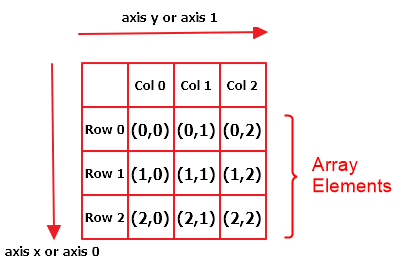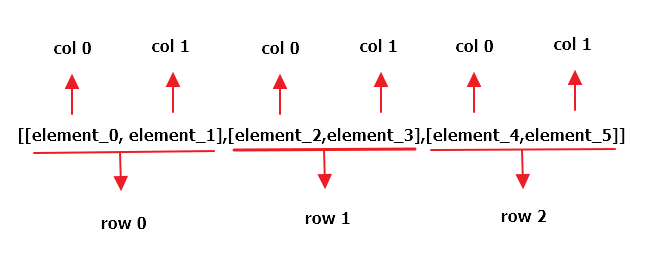# How To Create a Two Dimensional Array in Python?

[toc]

## Introduction

Today we can see 2D arrays used everywhere. As simple examples – chessboard, eggs arranged in a rectangular egg-holder are 2D arrays. In the world of computers, we use 2D arrays everywhere. 2D arrays are used in Image processing, Surveys, Speech Processing, Geoscience, Tables, Databases, Graphs, etc.

What is a Dimension in an array? Dimensions refer to a single level of depth in an array.

• 0D array is an array that has a single element. Simply put, each value in any array is a 0-D array.
• In a 1D array, the array will have just one dimension. That is, by adding the elements or by removing the elements, the array grows or shrinks just vertically. Only one index is used to access the elements of this array.

Note: Nested Arrays are arrays that have another array(s) as their elements.

A 2 Dimensional array is an array of arrays (nested array) that has two dimensions. 2D arrays can grow or shrink vertically as well as horizontally. It can be represented as rows and columns. A grid or matrix is an example of a 2D array. Every element is accessed using two indices, one corresponding to the row and another corresponding to the column. Refer to the below example.

Here’s a diagrammatic representation of a matrix (2D array) in Python that illustrates how they are indexed in Python:In this tutorial, you will learn the different ways of creating a 2D array.

## Method 1: Using Nested Lists

If you want to create an empty 2D array without using any external libraries, you can use nested lists. In simple words, a list of lists is a 2D array.

Example:

```arr_2d=[[1,2],[3,4],[5,6]]
print(arr_2d)

# [[1, 2], [3, 4], [5, 6]]```

Visualization: Consider the following 2D array.

[element_0  element_1
element_2  element_3
element_4  element_5]

The above 2D array can be represented with the help of a nested list in Python. Follow the illustration given below to visualize how its cells are indexed in Python:Now that you have a clear picture of how nested lists can be used to denote a 2D array in Python, let’s find out how we can create an empty 2D array (loaded with zeros), and then we shall learn how we can assign values to this array.

### Creating a 2D Array Loaded with Zeros

Using a For Loop:

To create/initialize an empty 2D array loaded with zeros, for every occurrence of a row, we fill all the column elements and append that to the row.

Approach: We first create a sub-list representing all the column values and append it to the outer list as shown below:

```for x in range(number_of_rows):
column_elements=[]
for y in range(number_of_columns):
# Enter the all the column values
column_elements.append(0)
#Append the column to the array.
arr_2d.append(column_elements)```

Example: In the following example you will learn to create a 2D array with 3 rows and 2 columns.

```number_of_rows = 3
number_of_columns = 2
arr_2d=[]
for x in range(number_of_rows):
column_elements=[]
for y in range(number_of_columns):
# Enter the all the values w.r.t to a particular column
column_elements.append(0)
#Append the column to the array.
arr_2d.append(column_elements)

print(arr_2d)```

Output:

``[[0, 0], [0, 0], [0, 0]]``

Using a List Comprehension:

Initializing arrays loaded with zeros is not such a long task if you know how list comprehensions work in Python. Using a list comprehension, you can replicate the above snippet in a single line of code as shown below:

`array_2d = [[0 for x in range(number_of_rows)] for y in range(number_of_columns)]`

Example:

```array_2d = [[0 for x in range(2)] for y in range(3)]
print(array_2d)

# [[0, 0], [0, 0], [0, 0]]
```

### Assigning Values to The 2D Array

Once the array has been initialized, assigning values is a cakewalk as you simply have to use proper indexing (rows and columns) to assign the values to the respective cells.

`Syntax: array_2d[row_number][column_number]= element`

Example: To assign values to all the elements of the first row, use the following syntax:

```array_2d=1
array_2d=2```

## Method 2: Using Dictionaries

Another way of creating 2D arrays in Python without using an external module is to use Python dictionaries. Dictionary acts as a placeholder to contain all the elements of the array.

✉️️Note: This approach is best suited only when you need to have a separate container to hold the values and the cells of the 2D array. If you have many operations to be performed on the array, this can get complicated, and it is not recommended in such cases.

Example: We are creating an empty dictionary array_2d and then filling in values.

```# Create an empty dictionary
array_2d = {}
# Assigning values:
array_2d[0, 0] = 1
array_2d[0, 1] = 2
array_2d[0, 2] = 3
array_2d[1, 0] = 4
array_2d[1, 1] = 5
array_2d[1, 2] = 6

# Segregating values w.r.t keys for convenience
array_2d['row_1'] = [array_2d[0, 0], array_2d[0, 1], array_2d[0, 2]]
array_2d['row_2'] = [array_2d[1, 0], array_2d[1, 1], array_2d[1, 2]]

# printing the 2D array:
for i in array_2d:
if type(array_2d[i]) is list:
print(array_2d[i])```

Output:

``````[1, 2, 3]
[4, 5, 6]``````

Explanation: In the above example, initially, we assigned the values to respective cells of the 2D array such the row and column index represent the keys of the dictionaries, and the values in each cell of the 2D array are denoted by the values associated with each key of the dictionary. For our convenience, we stored each row of the array in the form of a list within separate keys by combining the previously assigned keys within separate lists that serve as values to these keys. Finally, we print the array with the help of a` for loop` by simply displaying the keys of the dictionary which are storing the lists, i.e., the individual rows of the 2D array.

## Method 3: Using NumPy

The most convenient way of working with arrays in Python is to use Python’s Numpy library.

Python has this amazing module named `NumPy` that can be used to create multidimensional arrays. `Numpy` is specifically designed to work with arrays in Python. This library supports a lot of functions that can be used to perform operations on the array like matrix multiplication, transpose, matrix addition, etc. In fact, `Numpy` has a whole submodule that is dedicated towards matrices known as `numpy.mat`

Since Numpy is an external library, you have to install it before you can use it. To do so, use the following command on your terminal: pip install numpy

Once installed, you can import the Numpy module and use its functions and modules to work with multidimensional arrays.

### Creating 2D Arrays Loaded with Zeros

To create a 2D array loaded with zeros, you can simply use the numpy.zeros() method. This is what the official Numpy documentation states about the numpy.zeros() method.

``A Simple Syntax: arr=np.zeros((number_of_rows,number_of_columns))``

Example: To create an array with 3 rows and 2 columns

```import numpy as np

number_of_rows = 3
number_of_columns = 2

arr = np.zeros((number_of_rows, number_of_columns))
print(arr)```

Output:

``````[[0. 0.]
[0. 0.]
[0. 0.]]``````

If you have created an empty numpy array with zeros, here’s how you can use it later to assign values:

```import numpy as np

arr = np.zeros((3, 2))

arr[0, 0] = 1
arr[0, 1] = 2
arr[1, 0] = 3
arr[1, 1] = 4
arr[2, 0] = 5
arr[2, 1] = 6

print(arr)```

Output:

``````[[1. 2.]
[3. 4.]
[5. 6.]]``````

### Creating a 2D Array with Numpy

To create a numpy array object, you have to use the `numpy.array()` method.

```import numpy as np

arr = np.array([[1, 2, 3], [4, 5, 6], [7, 8, 9]])
print(arr)```

Output:

``````[[1 2 3]
[4 5 6]
[7 8 9]]``````

Note: A `Numpy` array object is known as `ndarray`.

### Bonus: Converting a List to NumPy Array

One way to convert the list to a numpy array is to just pass it within the `numpy.array()` method. Bt what if you have a simple list and you want to convert it into a 2D array??

Numpy once again has the solution to your problem as you can use the `numpy.arrange()` method to reshape a list into a 2D array.

Example: Let’s convert the list li = [1,2,3,4,5,6,7,8,9] to a n*n 2D array.

```import numpy as np

li = [1, 2, 3, 4, 5, 6, 7, 8, 9]
arr = np.array(li)
print(arr.reshape(3, 3))```

Output:

``````[[1 2 3]
[4 5 6]
[7 8 9]]``````

Explanation: Convert the list into a numpy array object with the help of the `np.array()` method and then use the `reshape()` method to convert this array to a 2D array.

Can We Reshape Into any Shape?
Yes, you can reshape an array in any shape as long as the elements present in a given array are equal to and can properly fit to the dimension of the array that you want to create. For example, you can easily reshape a 9 elements 1D array into 3 elements in 3 rows 2D array. However, you cannot reshape it to a 3 elements 2 rows 2D array, and it will throw a `ValueError`.

## Conclusion

In this tutorial, we have covered some of the basic ways to create a 2D array in Python. We hope this has been informative. Please stay tuned and subscribe for more such tips and tricks.

Post Credits: Shubham Sayon and Anusha Pai

## Programmer Humor

👱‍♀️ Programmer 1: We have a problem
🧔‍♂️ Programmer 2: Let’s use RegEx!
👱‍♀️ Programmer 1: Now we have two problems

… yet – you can easily reduce the two problems to zero as you polish your “RegEx Superpower in Python“. 🙂